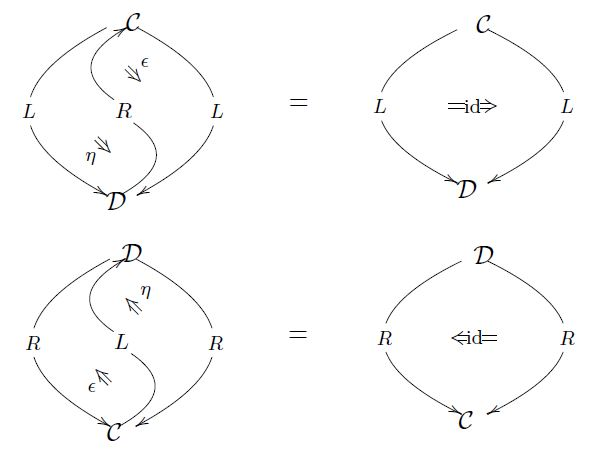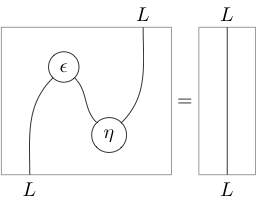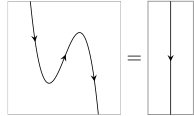# nLab triangle identities

Contents

category theory

## Applications

#### 2-Category theory

2-category theory

Definitions

Transfors between 2-categories

Morphisms in 2-categories

Structures in 2-categories

Limits in 2-categories

Structures on 2-categories

# Contents

## Idea

The triangle identities or zigzag identities are identities characterized by the unit and counit of an adjunction, such as a pair of adjoint functors. These identities define, equivalently, the nature of adjunction (this prop.).

## Statement

Consider:

1. $\mathcal{C}, \mathcal{D}$ a pair of categories, or, generally, of objects in a given 2-category;

2. $L \colon \mathcal{C} \to \mathcal{D}$ and $R \colon \mathcal{D} \to \mathcal{C}$ a pair of functors between these, or generally 1-morphisms in the ambient 2-category;

3. $\eta \colon id_{\mathcal{C}} \Rightarrow R \circ L$ and $\epsilon \colon L \circ R \Rightarrow id_{\mathcal{D}}$ two natural transformations or, generally 2-morphisms.

This data is called an pair of adjoint functors (generally: an adjunction) if the triangle identities are satisfied, which may be expressed in any of the following equivalent ways:

$\,$

### As equations

As equations, the triangle identities read

$\big( \epsilon L \big) \circ \big( L \eta \big) \;=\; id_L$
$\big( R \epsilon \big) \circ \big( \eta R \big) \;=\; id_R$

Here juxtaposition denotes the whiskering operation of 1-morphisms on 2-morphisms, as made more manifest in the diagrammatic unravelling of these expressions:

### As diagrams

In terms of diagrams in the functor categories this means

$L \overset{\;\;L\eta\;\;}{\Rightarrow} L R L \overset{\;\;\epsilon L\;\;}{\Rightarrow} L \;\; = \;\; L \overset{\;\;id_L\;\;}{\Rightarrow} L$

and

$R \overset{\;\;\eta R\;\;}{\Rightarrow} R L R \overset{\;\;R\epsilon\;\;}{\Rightarrow} R \;\; = \;\; R \overset{\;\;id_R\;\;}{\Rightarrow} R$

In terms of diagrams of 2-morphisms in the ambient 2-category, this looks as follows:

where on the right the identity 2-morphisms are left notationally implicit.

If we leave the identity 1-morphisms on the left notationally implicit, then we get the following suggestive form of the triangle identities:(taken from geometry of physics – categories and toposes).

### As string diagrams

As string diagrams, the triangle identities appear as the action of “pulling zigzags straight” (hence the name):With labels left implicit, this notation becomes very economical:,.

Textbook accounts include

See the references at category theory for more.